# Online geocoding in R using the NYS GIS server

Previously I wrote a post on using the NYS ESRI geocoding server in python. I recently wrote a function in R to do the same. The base url server has changed since I wrote the Python post, but it is easy to update that (the JSON returned doesn’t change.) This should also be simple to update for other ESRI servers, just change the `base` variable in the first function. This uses the httr package to get the url and the jsonlite package to parse the response.

``````#################################################################
#Functions for geocoding using online NYS GIS Esri API, https://gis.ny.gov/
library(httr)
library(jsonlite)

#getting a single address, WKID 4326 is WGS 1984, so returns lat/lon
dat <- fromJSON(content(soup,as='text'),simplifyVector=TRUE)\$candidates
return(dat)
}
#looping over a vector of addresses, parsing, and returning a data frame
#make empy matrix
MyDat <- data.frame(matrix(nrow=l,ncol=3))
for (i in 1:l){
if (length(x) > 0){
MyDat[i,1] <- x[,1]
MyDat[i,2] <- x[,2]
MyDat[i,3] <- x[,2]
}
}
return(MyDat)
}
#################################################################``````

The first function takes a single address, gets and parses the returning JSON. The second function loops over a list of addresses and returns a data frame with the original addresses, the matched address, and the lat/lon coordinates. I use a loop instead of an apply type function because with the web server you really shouldn’t submit large jobs that it would take along time anyway. The NYS server is free and has no 2,500 limit, but I wouldn’t submit jobs much bigger than that though.

``````AddList <- c("100 Washington Ave, Albany, NY","100 Washington Ave Ext, Albany, NY",
"421 New Karner Rd., Albany, NY","Washington Ave. and Lark St., Albany, NY","poop")

We can compare these to what the google geocoding api returns (using the ggmap package):

``````library(ggmap)

And we can see that the nonsense "poop" address was actually geocoded! See some similar related funny results from the google maps geocoding via StackMaps.

We can also see some confusion between Washington Ave. Ext as well. The NYS online server should theoretically have more up to date data than Google, but as above shows it is not always better. To do geocoding well takes some serious time to examine the initial addresses and the resulting coordinates in my experience.

To calculate the great circle distance between the coordinates we can use the `spDists` function in the sp library.

``````library(sp)
longlat=TRUE,diagonal=TRUE) #distance in kilometers``````

But really, we should just project the data and calculate the Euclidean distance (see the proj4 library). Note that using the law of cosines is typically not recommended for very small distances, so the last distance is suspect. (For reference I point to some resources and analysis showing how to calculate great circle distances in SPSS on Nabble recently.)

# Custom square root scale (with negative values) in ggplot2 (R)

My prior rootogram post Jon Peck made the astute comment that rootograms typically are plotted on a square root scale. (Which should of been obvious to me given the name!) The reason for a square root scale for rootograms is visualization purposes, the square root scale gives more weight to values nearby 0 and shrinks values farther away from 0.

SPSS can not have negative values on a square root scale, but you can make a custom scale using ggplot2 and the scales package in R for this purpose. Here I just mainly replicated this short post by Paul Hiemstra.

So in R, first we load the `scales` and the `ggplot2` package, and then create our custom scale function. Obviously the square root of a negative value is not defined for real numbers, so what we do is make a custom square root function. The function simply takes the square root of the absolute value, and then multiplies by the sign of the original value. This function I name `S_sqrt` (for signed square root). We also make its inverse function, which is named `IS_sqrt`. Finally I make a third function, `S_sqrt_trans`, which is the one used by the scales package.

``````library(scales)
library(ggplot2)

S_sqrt <- function(x){sign(x)*sqrt(abs(x))}
IS_sqrt <- function(x){x^2*sign(x)}
S_sqrt_trans <- function() trans_new("S_sqrt",S_sqrt,IS_sqrt)``````

Here is a quick example data set in R to work with.

``````#rootogram example, see http://stats.stackexchange.com/q/140473/1036
MyText <- textConnection("
Dist Val1 Val2
1 0.03 0.04
2 0.12 0.15
3 0.45 0.50
4 0.30 0.24
5 0.09 0.04
6 0.05 0.02
7 0.01 0.01
")
MyData\$Hang <- MyData\$Val1 - MyData\$Val2``````

And now we can make our plots in `ggplot2`. First the linear scale, and second update our plot to the custom square root scale.

``````p <- ggplot(data=MyData, aes(x = as.factor(Dist), ymin=Hang, ymax=Val1)) +
geom_hline(aes(yintercept=0)) + geom_linerange(size=5) + theme_bw()
p

p2 <- p + scale_y_continuous(trans="S_sqrt",breaks=seq(-0.1,0.5,0.05), name="Density")
p2``````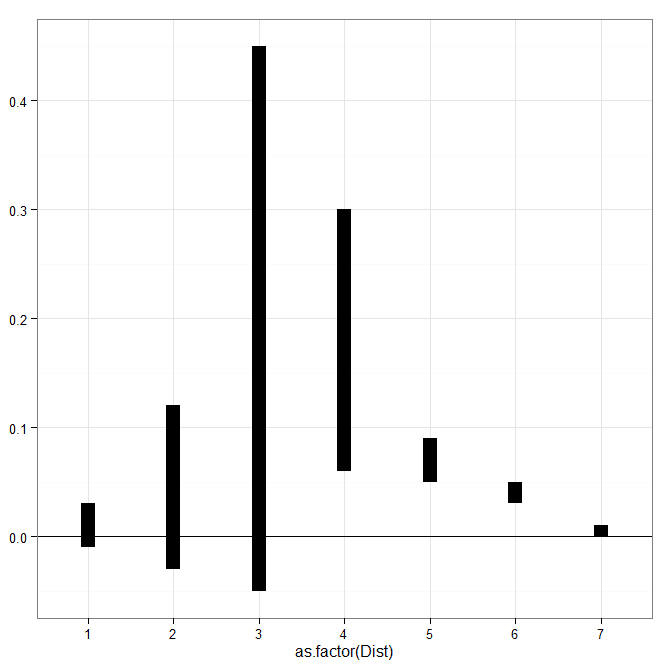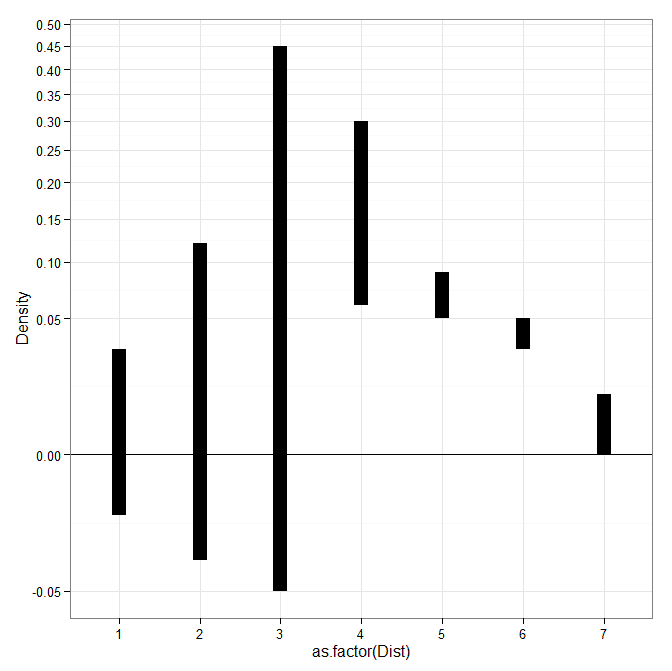# Venn diagrams in R (with some discussion!)

The other day I had a set of three separate categories of binary data that I wanted to visualize with a Venn diagram (or a Euler) diagram of their intersections. I used the `venneuler` R package and it worked out pretty well.

``````library(venneuler)
MyVenn <- venneuler(c(A=74344,B=33197,C=26464,D=148531,"A&B"=11797,
"A&C"=9004,"B&C"=6056,"A&B&C"=2172,"A&D"=0,"A&D"=0,"B&D"=0,"C&D"=0))
MyVenn\$labels <- c("A\n22","B\n7","C\n5","D\n58")
plot(MyVenn)
text(0.59,0.52,"1")
text(0.535,0.51,"3")
text(0.60,0.57,"2")
text(0.64,0.48,"4") ``````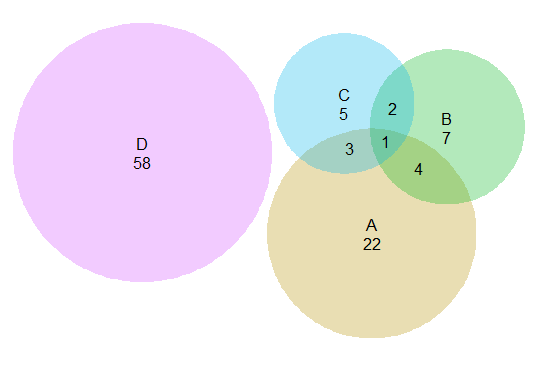Some digging around on this topic though I came across some pretty interesting discussion, in particular a graph makeover of a set of autism diagnoses, see:

for background. Below is a recreated image of the original Venn diagram under discussion (from Kosara’s American Scientist article.)Applying this example to the `venneuler` library did not work out so well.

``````MyVenn2 <- venneuler(c(A=111,B=65,C=94,"A&B"=62,"A&C"=77,"B&C"=52,"A&B&C"=51))
plot(MyVenn2)``````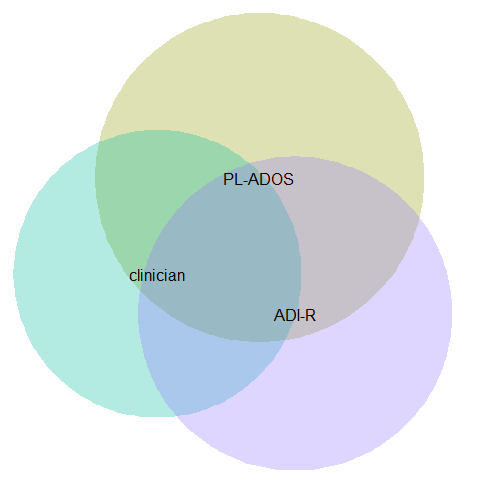Basically there is a limit on the size the intersections can be with the circles, and here the intersection of all three sets is very large, so there is no feasible solution for this example.

This is alittle bit different situation than typical for Venn diagrams though. Typically these charts all one is interested in is the overlaps between each set. But the autism graph that is secondary. What they were really interested in was the sensitivity of the different diagnostic measures (i.e. percentage identifying true positives), and to see if any particular combination had the greatest sensitivity. Although Kosara in his blog post says that all of the redesigns are better than the original I don’t entirely agree, I think Kosara’s version of the Venn diagram with the text labels does a pretty good job, although I think Kosara’s table is sufficient as well. (Kosara’s recreated graph has better labelling than the original Venn diagram, mainly by increasing the relative font size.)

For the autism graph there are basically two over-arching goals:

• identifying the percent within arbitrary multiple intersections
• keeping in mind the baseline N for each of the arbitrary sets

It is not immediately visually obvious, but IMO it is not that hard to arbitrarily collapse different categories in the original Venn diagram and make some rough judgements about the sensitivity. To me the first thing I look at is the center piece, see it is quite a high percentage, and then look to see if I can make any other arbitrary categories to improve upon the sensitivity of all three tests together. All others are either very small baselines or do not improve the percentage, so I conclude that all three combined likely have the most sensitivity. You may also see that the clinicians are quite high for each intersection, so it is questionable whether the two other diagnostics offer any significant improvement over just the clinicians judgement, but many of the clinician sets have quite small N’s, so I don’t put as much stock in that.

Another way to put it is if we think of the original Venn diagram as a graphical table I think it does a pretty good job. The circles and the intersections are a lie factor in the graph, in that their areas do not represent the baseline rates, but it is an intuitive way to lay out the textual categories, and only takes a little work to digest the material. Kosara’s sorted table does a nice job of this as well, but it is easier to ad-hoc combine categories in the Venn diagram than in table rows that are not adjacent. Visually the information does not pop out at you, like a functional relationship in a scatterplot, but the Venn diagram has the ingredients that allow you to drill down and estimate the information you are looking for. Being able to combine arbitrary categories is the key here, and I don’t think any of the other graphical representations allow one to do that very easily.

I thought a useful redesign would be to keep the Venn theme, but have the repeated structures show the base rate via Isotype like recurring graphs. Some of this is motivated by using such diagrams in interpreting statistics (see this post by David Spieghalter for one example, the work of Gerd Gigenzer is relevant as well). I was not able make a nice set of contained glyphs though. Here is a start of what I am talking about, I just exported the R graph into Inkscape and superimposed a bunch of rectangles.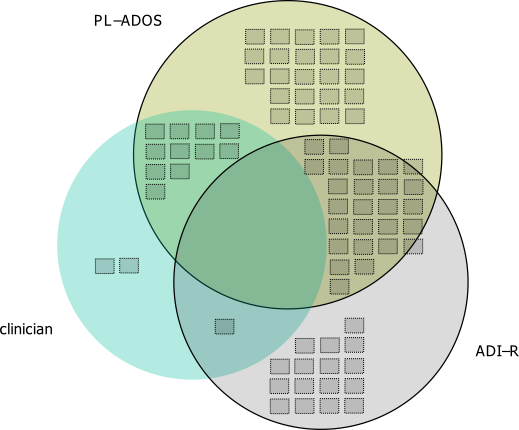This does not visualize the percentage, but one way to do that would be to color or otherwise distinguish the blocks in a certain way. Also I gave up before I finished the intersecting middle piece, and I would need to make the boxes a bit smaller to be able to squeeze it in. I think this idea could be made to work, but this particular example making the Venn even approximately proportional is impossible, and so sticking with the non-proportional Venn diagram and just saying it is not proportional is maybe less likely to be misleading.

I think the idea of using Isotype like repeated structures though could be a generally good idea. Even when the circles can be made to have the areas of the intersection exact, it is still hard to visually gauge the size of circles (rectangles are easier). So the multiple repeated pixels may be more useful anyway, and putting them inside of the circles still allows the arbitrary collapsing of different intersections while still being able to approximately gauge base rates.

# Transforming KDE estimates from Logistic to Probability Scale in R

The other day I had estimates from several logistic regression models, and I wanted to superimpose the univariate KDE’s of the predictions. The outcome was fairly rare, so the predictions were bunched up at the lower end of the probability scale, and the default kernel density estimates on the probability scale smeared too much of the probability outside of the range.

It is a general problem with KDE estimates, and there are two general ways to solve it:

• truncate the KDE and then reweight the points near the edge (example)
• estimate the KDE on some other scale that does not have a restricted domain, and then transform the density back to the domain of interest (example)

The first is basically the same as edge correction in spatial statistics, just in one dimension instead of the two. Here I will show how to do the second in R, mapping items on the logistic scale to the probability scale. The second linked CV post shows how to do this when using the log transformation, and here I will show the same with mapping logistic estimates (e.g. from the output of a logistic regression model). This requires the data to not have any values at 0 or 1 on the probability scale, because these will map to negative and positive infinity on the logistic scale.

In R, first define the logit function as `log(p/(1-p)` and the logistic function as `1/(1+exp(-x))` for use later:

``````logistic <- function(x){1/(1+exp(-x))}
logit <- function(x){log(x/(1-x))}``````

We can generate some fake data that might look like output from a logistic regression model and calculate the density object.

``````set.seed(10)
x <- rnorm(100,0,0.5)
l <- density(x)  #calculate density on logit scale``````

This blog post goes through the necessary math, but in a nut shell you can’t simply just transform the density estimate using the same function, you need to apply an additional transformation (referred to as the Jacobian). So here is an example transforming the density estimate from the logistic scale, `l` above, to the probability scale.

``````px <- logistic(l\$x)  #transform density to probability scale
py <- l\$y/(px*(1-px))
plot(px,py,type='l')``````

To make sure that the area does sum to one, we can superimpose the density calculated on the data transformed to the probability scale. In this example of fake data the two are pretty much identical. (Black line is my transformed density, and the red is the density estimate based on the probability data.)

``````dp <- density(logistic(x)) #density on the probability values to begin with
lines(dp\$x,dp\$y,col='red')``````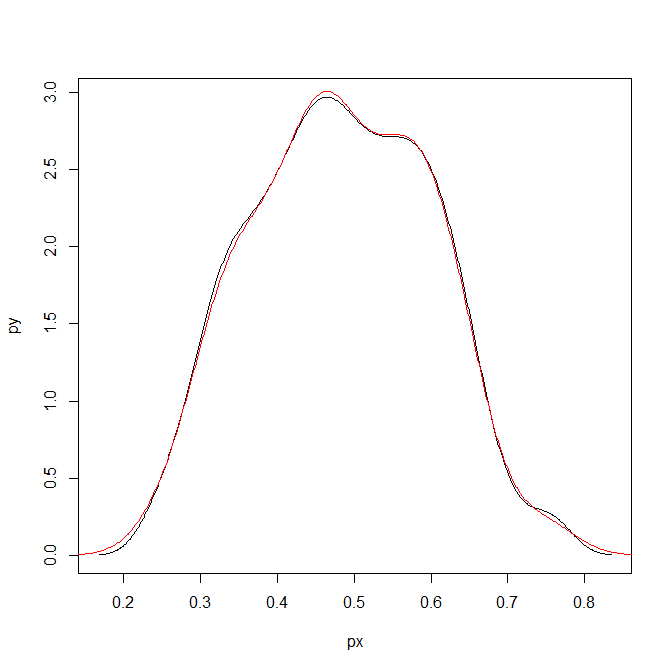Here is a helper function, `denLogistic`, to do this in the future, which simply takes the data (on the logistic scale) and returns a density object modified to the probability scale.

``````logistic <- function(x){1/(1+exp(-x))}
logit <- function(x){log(x/(1-x))}
denLogistic <- function(x){
d <- density(x)
d\$x <- logistic(d\$x)
d\$y <- d\$y/(d\$x*(1-d\$x))
d\$call <- 'Logistic Density Transformed to Probability Scale'
d\$bw <- paste0(signif(d\$bw,4)," (on Logistic scale)")
return(d)
}``````

In cases where more of the probability density is smeared beyond 0-1 on the probability scale, the logistic density estimate will look different. Here is an example with a wider variance and more predictions near zero, so the two estimates differ by a larger amount.

``````lP <- rnorm(100,-0.9,1)
test <- denLogistic(lP)
plot(test)
lines(density(logistic(lP)),col='red')``````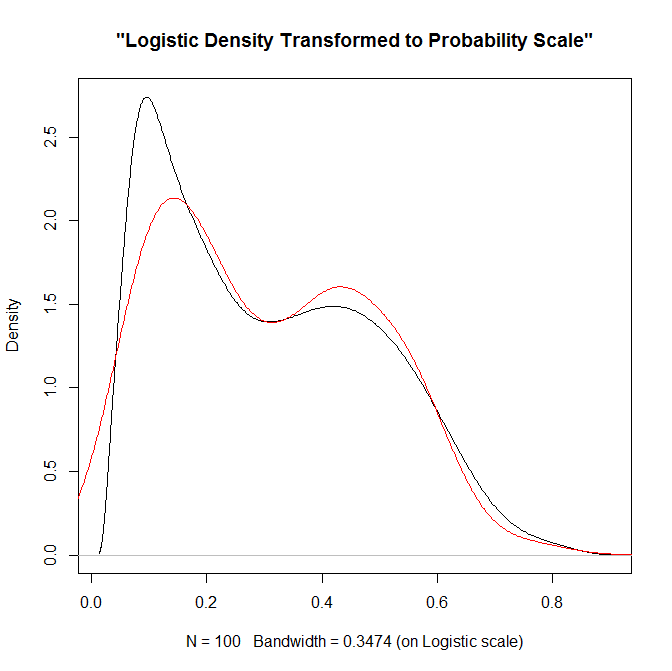Again, this works well for data on the probability scale that can not be exactly zero or one. If you have data like that, the edge correction type KDE estimators are better suited.

# Translating between the dispersion term in a negative binomial regression and random variables in SPSS

NOTE!! – when I initially posted this I was incorrect, I thought SPSS listed the dispersion term in the form of `Var(x) = mean + mean*dispersion`. But I was wrong, and it is `Var(x) = 1 + mean*dispersion` (the same as Stata’s, what Cameron and Trivedi call the NB2 model, as cited in the Long and Freese Stata book for categorical variables.) The simulation in the original post worked out because my example I used the mean as 1, here I update it to have a mean of 2 to show the calculations are correct. (Also note that this parametrization is equivalent to `Var(x) = mean*(1 + mean*dispersion)`, see Stata’s help for nbreg.)

When estimating a negative binomial regression equation in SPSS, it returns the dispersion parameter in the form of:

``Var(x) = 1 + mean*dispersion``

When generating random variables from the negative binomial distribution, SPSS does not take the parameters like this, but the more usual N trials with P successes. Stealing a bit from the R documentation for `dnbinom`, I was able to translate between the two with just a tedious set of algebra. So with our original distribution being:

``````Mean = mu
Variance = 1 + mu*a``````

R has an alternative representation closer to SPSS’s based on:

``````Mean = mu
Variance = mu + mu^2/x``````

Some tedious algebra will reveal that in this notation `x = mu^2/(1 - mu + a*mu)` (note to future self, using Solve in Wolfram Alpha could have saved some time, paper and ink). Also, R’s help for `dbinom` states that in the original N and P notation that `p = x/(x + mu)`. So here with `mu` and `a` (again `a` is the dispersion term as reported by GENLIN in SPSS) we can solve for `p`.

``````x = mu^2/(1 - mu + a*mu)
p = x/(x + mu)``````

And since `p` is solved, R lists the mean of the distribution in the N and P notation as:

``n*(1-p)/p = mu``

So with `p` solved we can figure out N as equal to:

``mu*p/(1-p) = n``

So to reiterate, if you have a mean of 2 and dispersion parameter of 4, the resultant N and P notation would be:

``````mu = 2
a = 4
x = mu^2/(1 - mu + a*mu) = 2^2/(1 - 2 + 4*2) = 4/7
p = x/(x + mu) = (4/7)/(4/7 + 2) = 2/9
n = mu*p/(1-p) = 2*(2/9)/(7/9) = 4/7``````

Here we can see that in the N and P notation the similar negative binomial model results in a fractional number of successes, which might be a surprising result for some that it is even a possibility. (There is likely an easier way to do this translation, but forgive me I am not a mathematician!)

Now we would be finished, but unfortunately SPSS’s negative binomial random functions only take integer values and do not take values of N less than 1 (R’s `dnbinom` does). So we have to do another translation of the N and P notation to the gamma distribution to be able to draw random numbers in SPSS. Another representation of the negative binomial model is a mixture of Poisson distributions, with the distribution of the mixtures being from a gamma distribution. Wikipedia lists a translation from the N and P notation to a gamma with shape = N and scale = P/(1-P).

So I wrapped these computations up in an SPSS macros that takes the mean and the dispersion parameter, calculates N and P under the hood, and then draws a random variable from the associated negative binomial distribution.

``````DEFINE !NegBinRV (mu = !TOKENS(1)
/disp = !TOKENS(1)
/out = !TOKENS(1) )
COMPUTE #x = !mu**2/(1 - !mu + !disp*!mu).
COMPUTE #p = #x / (#x + !mu).
COMPUTE #n = !mu*#p/(1 - #p).
COMPUTE #G = RV.GAMMA(#n,#p/(1 - #p)).
COMPUTE !Out = RV.POISSON(#G).
FORMATS !Out (F5.0).
!ENDDEFINE.``````

I am not sure if it is possible to use this gamma representation and native SPSS functions to calculate the corresponding CDF and PDF of the negative binomial distribution. But we can use R to do that. Here is an example of keeping the mean at 1 and varying the dispersion parameter between 0 and 5.

``````BEGIN PROGRAM R.
library(ggplot2)
x <- expand.grid(0:10,1:5)
names(x) <- c("Int","Disp")
mu <- 1
x\$PDF <- mapply(dnbinom, x=x\$Int, size=mu^2/(1 - mu + x\$Disp*mu), mu=mu)
t <- data.frame(cbind(0:10,rep(0,11),dpois(0:10,lambda=1)))
names(t) <- c("Int","Disp","PDF")
x <- rbind(t,x)
p <- ggplot(data = x, aes(x = Int, y = PDF, group = as.factor(Disp))) + geom_line()
p
#for the CDF
x\$CDF <- ave(x\$PDF, x\$Disp, FUN = cumsum)
END PROGRAM.``````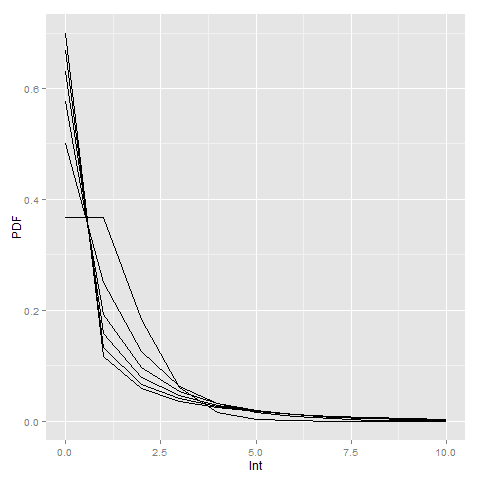Here you can see how the larger dispersion term can easily approximate the zero inflation typical in criminal justice data (see an applied example from my work). R will not take a dispersion parameter of zero in this notation (as the size would be divided by zero and not defined), so I just tacked on the Poisson distribution with a mean of zero.

Here is an example of generating random data from a negative binomial distribution with a mean of 2 and a dispersion parameter of 4. I then grab the PDF from R, and superimpose them both on a chart in SPSS (or perhaps I should call it a PMF, since it only has support on integer values). You can see the simulation with 10,000 observations is a near perfect fit (so a good sign I did not make any mistakes!)

``````*Simulation In SPSS.
INPUT PROGRAM.
LOOP Id = 1 TO 10000.
END CASE.
END LOOP.
END FILE.
END INPUT PROGRAM.
DATASET NAME RandNB.

!NegBinRV mu = 2 disp = 4 out = NB.

*Making seperate R dataset of PDF.
BEGIN PROGRAM R.
mu <- 2
disp <- 4
x <- 0:11
pdf <- dnbinom(x=x,size=mu^2/(1 - mu + disp*mu),mu=mu)
pdf[max(x)+1] <- 1 - sum(pdf[-(max(x)+1)])
MyDf <- data.frame(cbind(x,pdf))
END PROGRAM.
EXECUTE.
STATS GET R FILE=* /GET DATAFRAME=MyDf DATASET=PDF_NB.
DATASET ACTIVATE PDF_NB.
FORMATS x (F2.0).
VALUE LABELS x 11 '11 or More'.

*Now superimposing bar plot and PDF from separate datasets.
DATASET ACTIVATE RandNB.
RECODE NB (11 THRU HIGHEST = 11)(ELSE = COPY) INTO NB_Cat.
FORMATS NB_Cat (F2.0).
VALUE LABELS NB_Cat 11 '11 or More'.

GGRAPH
/GRAPHDATASET NAME="Data" DATASET='RandNB' VARIABLES=NB_Cat[LEVEL=ORDINAL] COUNT()[name="COUNT"]
/GRAPHDATASET NAME="PDF" DATASET='PDF_NB' VARIABLES=x pdf
/GRAPHSPEC SOURCE=INLINE.
BEGIN GPL
SOURCE: Data=userSource(id("Data"))
DATA: NB_Cat=col(source(Data), name("NB_Cat"), unit.category())
DATA: COUNT=col(source(Data), name("COUNT"))
SOURCE: PDF=userSource(id("PDF"))
DATA: x=col(source(PDF), name("x"), unit.category())
DATA: den=col(source(PDF), name("pdf"))
TRANS: den_per = eval(den*100)
GUIDE: axis(dim(1))
GUIDE: axis(dim(2))
SCALE: linear(dim(2), include(0))
ELEMENT: interval(position(summary.percent(NB_Cat*COUNT)), shape.interior(shape.square))
ELEMENT: point(position(x*den_per), color.interior(color.black), size(size."8"))
END GPL.``````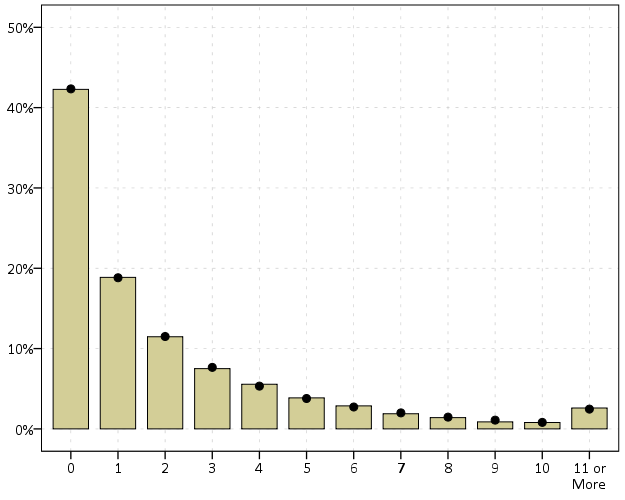# Estimating group based trajectory models using SPSS and R

For a project I have been estimating group based trajectory models for counts of crime at micro places. Synonymous with the trajectory models David Weisburd and colleagues estimated for street segments in Seattle. Here I will show how using SPSS and the R package `crimCV` one can estimate similar group based trajectory models. Here is the `crimCV` package help and here is a working paper by the authors on the methodology. The package specifically estimates a zero inflated poisson model with the options to make the 0-1 part and/or the count part have quadratic or cubic terms – and of course allows you specify the number of mixture groups to search for.

So first lets make a small set of fake data to work with. I will make 100 observations with 5 time points. The trajectories are three separate groups (with no zero inflation).

``````*Make Fake data.
SET SEED 10.
INPUT PROGRAM.
LOOP Id = 1 TO 100.
END CASE.
END LOOP.
END FILE.
END INPUT PROGRAM.
DATASET NAME OrigData.
*Make 3 fake trajectory profiles.
VECTOR Count(5,F3.0).
DO REPEAT Count = Count1 TO Count5 /#iter = 1 TO 5.
COMPUTE #i = #iter - 3.
COMPUTE #ii  = #i**2.
COMPUTE #iii = #i**3.
DO IF Id <= 30.
COMPUTE #P    = 10 + #i*0.3 + #ii*-0.1 + #iii*0.05.
COMPUTE Count = RV.POISSON(#P).
ELSE IF Id <=60.
COMPUTE #P    =  5 + #i*-0.8 + #ii*0.3 + #iii*0.05.
COMPUTE Count = RV.POISSON(#P).
ELSE.
COMPUTE #P    =  4 + #i*0.8 + #ii*-0.5 + #iii*0.
COMPUTE Count = RV.POISSON(#P).
END IF.
END REPEAT.
FORMATS Id Count1 TO Count5 (F3.0).
EXECUTE.``````

Note The `crimCV` package wants the data to be wide format for the models, that is each separate time point in its own column. Now we can call R code using `BEGIN PROGRAM R` to recreate the wide SPSS dataset in an R data frame.

``````*Recreate SPSS data in R data frame.
BEGIN PROGRAM R.
casedata <- spssdata.GetDataFromSPSS(variables=c("Id","Count1","Count2",
"Count3","Count4","Count5")) #grab data from SPSS
casedataMat <- as.matrix(casedata[,2:6]) #turn into matrix
#summary(casedata) #check contents
#casedataMat[1:10,1:5]
END PROGRAM.``````

Now to fit one model with 3 groups (without calculating the cross validation statistics) the code would be as simple as:

``````*Example estimating model with 3 groups and no CV.
BEGIN PROGRAM R.
library(crimCV)
crimCV(casedataMat,3,rcv=FALSE,dpolyp=3,dpolyl=3)
END PROGRAM.``````

But when we are estimating these group based trajectory models we don’t know the number of groups in advance. So typically one progressively fits more groups and then uses model selection criteria to pick the mixture solution that best fits the data. Here is a loop I created to successively estimate models with more groups and stuffs the models results in a list. It also makes a separate data frame that saves the model fit statistics, so you can see which solution fits the best (at least based on these statistics). Here I loop through estimates of 1 through 4 groups (this takes about 2 minutes in this example). Be warned – here are some bad programming practices in R (the for loops are defensible, but growing the lists within the loop is not – they are small though in my application and I am lazy).

``````*looping through models 1 through 4.
BEGIN PROGRAM R.
results <- c()  #initializing a set of empty lists to store the seperate models
measures <- data.frame(cbind(groups=c(),llike=c(),AIC=c(),BIC=c(),CVE=c())) #nicer dataframe to check out model
#model selection diagnostics
max <- 4 #this is the number of grouping solutions to check

#looping through models
for (i in 1:max){
model <- crimCV(casedataMat,i,rcv=TRUE,dpolyp=3,dpolyl=3)
results <- c(results, list(model))
measures <- rbind(measures,data.frame(cbind(groups=i,llike=model\$llike,
AIC=model\$AIC,BIC=model\$BIC,CVE=model\$cv)))
#save(measures,results,file=paste0("Traj",as.character(i),".RData")) #save result
}
#table of the model results
measures
END PROGRAM.``````

In my actual application the groups take a long time to estimate, so I have the commented line saving the resulting list in a file. Also if the model does not converge it breaks the loop. So here we see that the mixture with 3 groups is the best fit according to the CVE error, but the 2 group solution would be chosen by AIC or BIC criteria. Just for this tutorial I will stick with the 3 group solution. We can plot the predicted trajectories right within R by selecting the nested list.

``````*plot best fitting model.
BEGIN PROGRAM R.
plot(results[])
#getAnywhere(plot.dmZIPt) #this is the underlying code
END PROGRAM.``````

Now the particular object that stores the probabilities is within the `gwt` attribute, so we can transform this to a data frame, merge in the unique identifier, and then use the `STATS GET R` command to grab the resulting R data frame back into an SPSS dataset.

``````*Grab probabiltiies back SPSS dataset.
BEGIN PROGRAM R.
myModel <- results[] #grab model
myDF <- data.frame(myModel\$gwt) #probabilites into dataframe
myDF\$Id <- casedata\$Id #add in Id
END PROGRAM.
*back into SPSS dataset.
STATS GET R FILE=* /GET DATAFRAME=myDF DATASET=TrajProb.``````

Then we can merge this R data frame into SPSS. After that, we can classify the observations into groups based on the maximum posterior probability of belonging to a particular group.

``````*Merge into original dataset, and the assign groups.
DATASET ACTIVATE OrigData.
MATCH FILES FILE = *
/FILE = 'TrajProb'
/BY ID
/DROP row.names.
DATASET CLOSE TrajProb.
*Assign group based on highest prob.
*If tied last group wins.
VECTOR X = X1 TO X3.
COMPUTE #MaxProb = MAX(X1 TO X3).
LOOP #i = 1 TO 3.
IF X(#i) = #MaxProb Group = #i.
END LOOP.
FORMATS Group (F1.0).``````

Part of the motivation for doing this is not only to replicate some of the work of Weisburd and company, but that it has utility for identifying long term hot spots. Part of what Weisburd (and similarly I am) finding is that crime at small places is pretty stable over long periods of time. So we don’t need to make up to date hotspots to allocate police resources, but are probably better off looking at crime over much longer periods to identify places for targeted strategies. Trajectory models are a great tool to help identify those long term high crime places, same as geographic clustering is a great tool to help identify crime hot spots.

# Jittered scatterplots with 0-1 data

Scatterplots with discrete variables and many observations take some touches beyond the defaults to make them useful. Consider the case of a categorical outcome that can only take two values, 0 and 1. What happens when we plot this data against a continuous covariate with my default chart template in SPSS?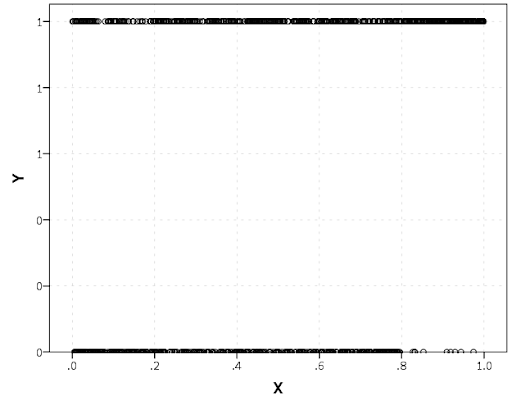Oh boy, that is not helpful. Here is the fake data I made and the `GGRAPH` code to make said chart.

``````*Inverse logit - see.
*https://andrewpwheeler.wordpress.com/2013/06/25/an-example-of-using-a-macro-to-make-a-custom-data-transformation-function-in-spss/.
DEFINE !INVLOGIT (!POSITIONAL  !ENCLOSE("(",")") )
1/(1 + EXP(-!1))
!ENDDEFINE.

SET SEED 5.
INPUT PROGRAM.
LOOP #i = 1 TO 1000.
COMPUTE X = RV.UNIFORM(0,1).
DO IF X <= 0.2.
COMPUTE YLin = -0.5 + 0.3*(X-0.1) - 4*((X-0.1)**2).
ELSE IF X > 0.2 AND X < 0.8.
COMPUTE YLin = 0 - 0.2*(X-0.5) + 2*((X-0.5)**2) - 4*((X-0.5)**3).
ELSE.
COMPUTE YLin = 3 + 3*(X - 0.9).
END IF.
COMPUTE #YLin = !INVLOGIT(YLin).
COMPUTE Y = RV.BERNOULLI(#YLin).
END CASE.
END LOOP.
END FILE.
END INPUT PROGRAM.
DATASET NAME NonLinLogit.
FORMATS Y (F1.0) X (F2.1).

*Original chart.
GGRAPH
/GRAPHDATASET NAME="graphdataset" VARIABLES=X Y
/GRAPHSPEC SOURCE=INLINE.
BEGIN GPL
SOURCE: s=userSource(id("graphdataset"))
DATA: X=col(source(s), name("X"))
DATA: Y=col(source(s), name("Y"))
GUIDE: axis(dim(1), label("X"))
GUIDE: axis(dim(2), label("Y"))
ELEMENT: point(position(X*Y))
END GPL.``````

So here we will do a few things to the chart to make it easier to interpret:

SPSS can jitter the points directly within `GGRAPH` code (see `point.jitter`), but here I jitter the data slightly myself a uniform amount. The extra aesthetic options for making points smaller and semi-transparent are at the end of the `ELEMENT` statement.

``````*Making a jittered chart.
COMPUTE YJitt = RV.UNIFORM(-0.04,0.04) + Y.
FORMATS Y YJitt (F1.0).
GGRAPH
/GRAPHDATASET NAME="graphdataset" VARIABLES=X Y YJitt
/GRAPHSPEC SOURCE=INLINE.
BEGIN GPL
SOURCE: s=userSource(id("graphdataset"))
DATA: X=col(source(s), name("X"))
DATA: Y=col(source(s), name("Y"))
DATA: YJitt=col(source(s), name("YJitt"))
GUIDE: axis(dim(1), label("X"))
GUIDE: axis(dim(2), label("Y"), delta(1), start(0))
SCALE: linear(dim(2), min(-0.05), max(1.05))
ELEMENT: point(position(X*YJitt), size(size."3"),
transparency.exterior(transparency."0.7"))
END GPL.``````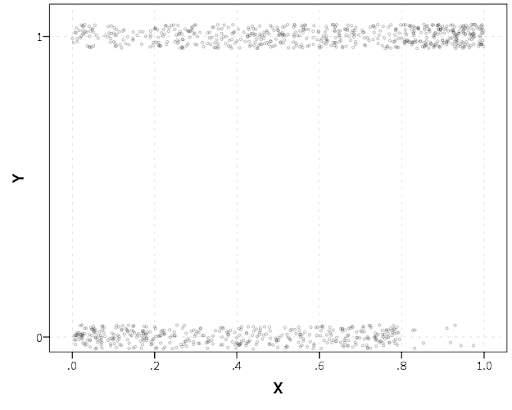If I made the Y axis categorical I would need to use `point.jitter` in the inline GPL code because SPSS will always force the categories to the same spot on the axis. But since I draw the Y axis as continuous here I can do the jittering myself.

A useful tool for exploratory data analysis is to add a smoothing term to plot – a local estimate of the mean at different locations of the X-axis. No binning necessary, here is an example using loess right within the `GGRAPH` call. The red line is the smoother, and the blue line is the actual proportion I generated the fake data from. It does a pretty good job of identifying the discontinuity at 0.8, but the change points earlier are not visible. Loess was originally meant for continuous data, but for exploratory analysis it works just fine on the 0-1 data here. See also `smooth.mean` for 0-1 data.

``````*Now adding in a smoother term.
COMPUTE ActualFunct = !INVLOGIT(YLin).
FORMATS Y YJitt ActualFunct (F2.1).
GGRAPH
/GRAPHDATASET NAME="graphdataset" VARIABLES=X Y YJitt ActualFunct
/GRAPHSPEC SOURCE=INLINE.
BEGIN GPL
SOURCE: s=userSource(id("graphdataset"))
DATA: X=col(source(s), name("X"))
DATA: Y=col(source(s), name("Y"))
DATA: YJitt=col(source(s), name("YJitt"))
DATA: ActualFunct=col(source(s), name("ActualFunct"))
GUIDE: axis(dim(1), label("X"))
GUIDE: axis(dim(2), label("Y"), delta(0.2), start(0))
SCALE: linear(dim(2), min(-0.05), max(1.05))
ELEMENT: point(position(X*YJitt), size(size."3"),
transparency.exterior(transparency."0.7"))
ELEMENT: line(position(smooth.loess(X*Y, proportion(0.2))), color(color.red))
ELEMENT: line(position(X*ActualFunct), color(color.blue))
END GPL.``````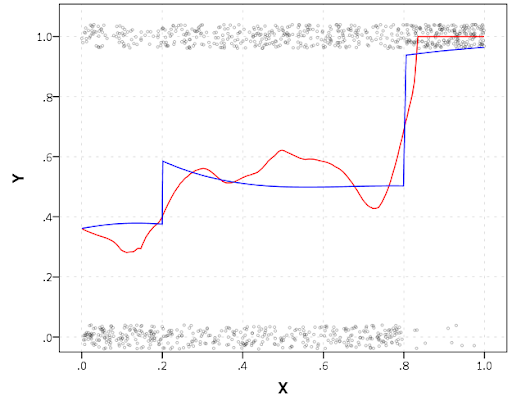SPSS’s default smoothing is alittle too smoothed for my taste, so I set the proportion of the X variable to use in estimating the mean within the `position` statement.

I wish SPSS had the ability to draw error bars around the smoothed means (you can draw them around the linear regression lines with quadratic or cubic polynomial terms, but not around the local estimates like `smooth.loess` or `smooth.mean`). I realize they are not well defined and rarely have coverage properties of typical regression estimators – but I rather have some idea about the error than no idea. Here is an example using the `ggplot2` library in R. Of course we can work the magic right within SPSS.

``````BEGIN PROGRAM R.
#Grab Data
casedata <- spssdata.GetDataFromSPSS(variables=c("Y","X"))
#ggplot smoothed version
library(ggplot2)
library(splines)
MyPlot <- ggplot(aes(x = X, y = Y), data = casedata) +
geom_jitter(position = position_jitter(height = .04, width = 0), alpha = 0.1, size = 2) +
stat_smooth(method="glm", family="binomial", formula = y ~ ns(x,5))
MyPlot
END PROGRAM.``````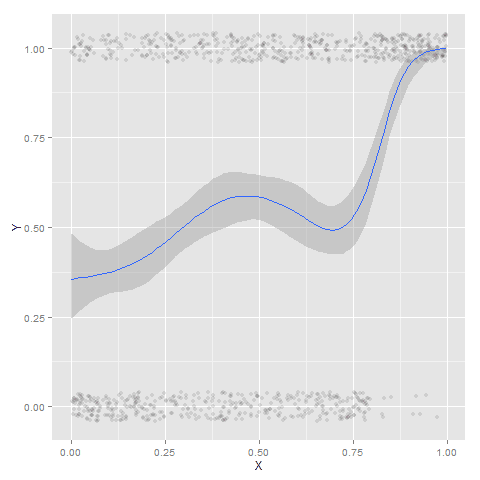To accomplish the same thing in SPSS you can estimate restricted cubic splines and then use any applicable regression procedure (e.g. `LOGISTIC`, `GENLIN`) and save the predicted values and confidence intervals. It is pretty easy to call the R code though!

I haven’t explored the automatic linear modelling, so let me know in the comments if there is a simply way right in SPSS to get explore such non-linear predictions.

# The mad scientist workflow: Some examples of using python and R within SPSS

I figured I would share some of the scripts I have been recently working on to produce a set of figures on a regular basis for reports. SPSS GGRAPH can not be directly parameterized within macro’s (at least without a lot of work – see a counter example of Marta Garcia-Granero’s macro for Kalbfleisch-Prentice 95%CI for survival), but can be called using python code. Jon Peck has some examples at the Developerworks blog, and here I will show some more! I am also going to show how to make some automated maps in R using the ggmap package, with which you can grab various basemap tiles from online and superimpose point data.

So lets provide some example data to work with.

``````DATA LIST FREE / Id (A1) Crime (A8) Hour (F2.0) Lon Lat (2F16.8).
BEGIN DATA
1 Robbery 18 -74.00548939   41.92961837
2 Robbery 19 -73.96800055   41.93152595
3 Robbery 19 -74.00755075   41.92996862
4 Burglary 11 -74.01183343  41.92925202
5 Burglary 12 -74.00708100  41.93262613
6 Burglary 14 -74.00923393  41.92667552
7 Burglary 12 -74.00263453  41.93267197
END DATA.
DATASET NAME Crimes.``````

And now let’s say you want to make a graph of the hours of the day that Robberies occur in. Many small to mid-range police departments have few serious crimes when examining over shorter time spans (like a week or a month). So one type of chart I like to use are histogram like dot plots. Here is an example for the entire dataset in question.

``````GGRAPH
/GRAPHDATASET NAME="graphdataset" VARIABLES=Hour MISSING=LISTWISE REPORTMISSING=NO
/GRAPHSPEC SOURCE=INLINE.
BEGIN GPL
PAGE: begin(scale(600px,300px))
SOURCE: s=userSource(id("graphdataset"))
DATA: Hour=col(source(s), name("Hour"))
TRANS: bottom=eval(0)
TRANS: top=eval(10)
TRANS: begin=eval(7)
TRANS: end=eval(14.5)
COORD: rect(dim(1,2))
GUIDE: axis(dim(1), label("Hour of Day"), delta(1))
GUIDE: axis(dim(2), null())
GUIDE: text.title(label("Crimes by Hour Reported"))
SCALE: linear(dim(1), min(1), max(22.5))
SCALE: linear(dim(2), min(0), max(3))
transparency.interior(transparency."0.5"), transparency.exterior(transparency."1"))
ELEMENT: point.dodge.asymmetric(position(bin.dot(Hour, dim(1), binWidth(1))), color.interior(color.darkgrey), size(size."30"))
PAGE: end()
END GPL.``````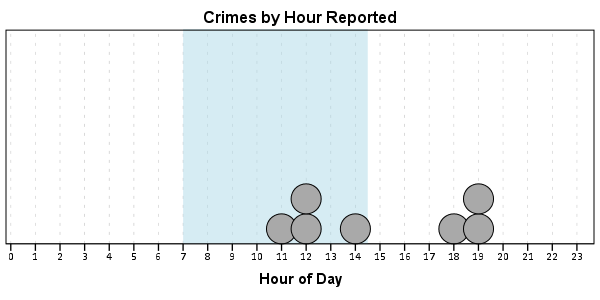One of the things I like to do though is to make charts for different subsets of the data. One way is to use `FILTER` to only plot a subset of the data, but a problem with this approach is the fixed aspects of the chart, like the title, do not change to reflect the current data. Here I will use `FILTER` in combination with a python `BEGIN PROGRAM ... END PROGRAM` block to grab the crime type to insert into the title of the graph.

``````COMPUTE Bur = (Crime = "Burglary").
FILTER BY Bur.
BEGIN PROGRAM.
import spss
MyVars = 
dataCursor=spss.Cursor(MyVars)
MyData=dataCursor.fetchone()
dataCursor.close()
CrimeType = MyData.strip()
print CrimeType
END PROGRAM.
FILTER OFF.``````

So when grabbing the SPSS case data, python respects the current `FILTER` on the dataset. First I set an array, `MyVars`, to grab the variables I want. Here I only want the `Crime` variable, which is the second variable in the dataset. Python’s arrays are indexed at zero, so I end up wanting the variable in the `` position. Then SPSS has a set of functions to grab data out of the active dataset using `spss.Cursor`. What I do is use the `fetchone()` object property to only grab the first row of data, and assign it the name `MyData`. Then after closing the cursor using `dataCursor.close()`, I access the string that is in the first location in the array `MyData` and use the `strip()` property to clean up trailing blanks in the string (see this example on Stackoverflow for where this was handy). When running the above code you can see that it prints `Burglary`, even though the burglary cases are not the first ones in the dataset. Now we can extend this example to insert the chart title and submit the GGRAPH syntax.

``````FILTER BY Bur.
BEGIN PROGRAM.
import spss
MyVars = 
dataCursor=spss.Cursor(MyVars)
MyData=dataCursor.fetchone()
dataCursor.close()
CrimeType = MyData.strip()
spss.Submit("""
GGRAPH
/GRAPHDATASET NAME="graphdataset" VARIABLES=Hour MISSING=LISTWISE REPORTMISSING=NO
/GRAPHSPEC SOURCE=INLINE.
BEGIN GPL
PAGE: begin(scale(600px,300px))
SOURCE: s=userSource(id("graphdataset"))
DATA: Hour=col(source(s), name("Hour"))
TRANS: bottom=eval(0)
TRANS: top=eval(10)
TRANS: begin=eval(7)
TRANS: end=eval(14.5)
COORD: rect(dim(1,2))
GUIDE: axis(dim(1), label("Hour of Day"), delta(1))
GUIDE: axis(dim(2), null())
GUIDE: text.title(label("%s by Hour Reported"))
SCALE: linear(dim(1), min(1), max(22.5))
SCALE: linear(dim(2), min(0), max(3))
transparency.interior(transparency."0.5"), transparency.exterior(transparency."1"))
ELEMENT: point.dodge.asymmetric(position(bin.dot(Hour, dim(1), binWidth(1))), color.interior(color.darkgrey), size(size."30"))
PAGE: end()
END GPL.
""" %(CrimeType))
END PROGRAM.
FILTER OFF.``````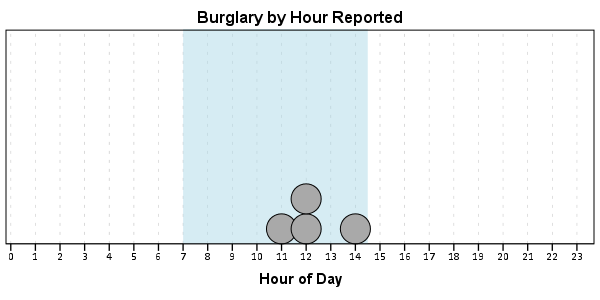You can do this same process for calling R commands, as again grabbing the case data from SPSS respects the current `FILTER` or (`TEMPORARY` + `SELECT IF`). One favorite of mine recently is to make automated maps using the `ggmap` library. Grabbing the online tiles makes my job of making a nice background much easier. Here is an example of grabbing case data from SPSS and placing the locations on the map (note this is made up data, these aren’t actual crime locations in Kingston!)

``````FILTER BY Bur.
BEGIN PROGRAM R.
#using ggmap to make a basemap
library(ggmap)
loc <- c(left = -74.04, bottom = 41.91, right = -73.960, top = 41.948)
KingstonBasemap <- get_map(location = loc, zoom = 14, maptype = "toner",
source = "stamen")

#setting styles for ggplot map
axisStyle <- theme(axis.text.y=element_blank(),axis.text.x=element_blank(),
axis.ticks=element_blank(),axis.title.x=element_blank(),
axis.title.y=element_blank()
)
titleStyle <- theme(plot.title = element_text(face="bold", size=25))

#now grabbing SPSS data
casedata <- spssdata.GetDataFromSPSS(variables=c("Id","Crime","Lon","Lat"))
TypeCrime <- as.character(casedata\$Crime) #grabs first case for chart title

#Data to put on the map
point <- geom_point(aes(x = Lon, y = Lat), data=casedata, shape=21, size = 9, fill = "Blue")
labels <- geom_text(aes(x = Lon, y = Lat, label = as.character(Id)), data=casedata,
hjust = -0.03, vjust = -0.8, size = 8, fontface=2, color="cornflowerblue"
)
title <- labs(title=paste0(TypeCrime," cases in March"))

#Putting all together
CrimeMap <- ggmap(KingstonBasemap) + point + labels + axisStyle + titleStyle + title
CrimeMap
END PROGRAM.
FILTER OFF.``````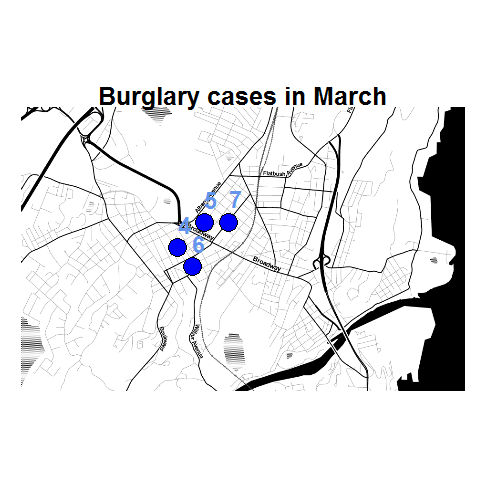To make the map look nice takes a bit of code, but all of the action with grabbing data from SPSS and setting the string I want to use in my chart title are in the two lines of code after the `#now grabbing SPSS data` comment. (Note I like using Stamen base maps as they allow one to grab non-square tiles. I typically like to use the terrain map – not because the terrain is necessary but just because I like the colors – but I’ve been having problems using the terrain tiles.)

Basically the set up I have now is to place some arbitrary code for graphs and maps in a separate syntax file. So all I need to do set the filter, use `INSERT`, and then turn the filter off. I can then add graphs for any subsets I am interested in. I have a separate look up table that stashes all the necessary metadata I want for use in the plots for whatever particular categories, which in addition to titles include other chart aesthetics like sizes for point elements or colors. In the future I will have to explore more options for using the `SPLIT FILE` facilities that both python and R offer when working with SPSS case data, but this is pretty simple and generalizes to non-overlapping groups as well.

# Avoid Dynamite Plots! Visualizing dot plots with super-imposed confidence intervals in SPSS and R

Over at the stats.se site I have come across a few questions demonstrating the power of utilizing dot plots to visualize experimental results.

Also some interesting discussion on what error bars to plot in similar experiments is in this question, Follow up: In a mixed within-between ANOVA plot estimated SEs or actual SEs?

Here I will give two examples utilizing SPSS and R to produce similar plots. I haven’t annotated the code that much, but if you need anything clarified on what the code is doing let me know in the comments. The data is taken from this question on the stats site.

# Citations of Interest to the Topic

## SPSS Code to generate below dot plot``````*******************************************************************************************. data list free /NegVPosA NegVNtA    PosVNegA    PosVNtA NtVNegA NtVPosA.
begin data
0.5 0.5 -0.4    0.8 -0.45   -0.3
0.25    0.7 -0.05   -0.35   0.7 0.75
0.8 0.75    0.65    0.9 -0.15   0
0.8 0.9 -0.95   -0.05   -0.1    -0.05
0.9 1   -0.15   -0.35   0.1 -0.85
0.8 0.8 0.35    0.75    -0.05   -0.2
0.95    0.25    -0.55   -0.3    0.15    0.3
1   1   0.3 0.65    -0.25   0.35
0.65    1   -0.4    0.25    0.3 -0.8
-0.15   0.05    -0.75   -0.15   -0.45   -0.1
0.3 0.6 -0.7    -0.2    -0.5    -0.8
0.85    0.45    0.2 -0.05   -0.45   -0.5
0.35    0.2 -0.6    -0.05   -0.3    -0.35
0.95    0.95    -0.4    0.55    -0.1    0.8
0.75    0.3 -0.05   -0.25   0.45    -0.45
1   0.9 0   0.5 -0.4    0.2
0.9 0.25    -0.25   0.15    -0.65   -0.7
0.7 0.6 -0.15   0.05    0   -0.3
0.8 0.15    -0.4    0.6 -0.05   -0.55
0.2 -0.05   -0.5    0.05    -0.5    0.3
end data.
dataset name dynamite.

*reshaping the data wide to long, to use conditions as factors in the plot.

varstocases
/make condition_score from NegVPosA to NtVPosA
/INDEX = condition (condition_score).

*dot plot, used dodge symmetric instead of jitter.
GGRAPH
/GRAPHDATASET dataset = dynamite NAME="graphdataset" VARIABLES=condition condition_score MISSING=LISTWISE
REPORTMISSING=NO
/GRAPHSPEC SOURCE=INLINE.
BEGIN GPL
SOURCE: s=userSource(id("graphdataset"))
DATA: condition=col(source(s), name("condition"), unit.category())
DATA: condition_score=col(source(s), name("condition_score"))
GUIDE: axis(dim(1), label("condition"))
GUIDE: axis(dim(2), label("condition_score"))
ELEMENT: point.dodge.symmetric(position(condition*condition_score))
END GPL.

*confidence interval plot.

*cant get gpl working (maybe it is because older version) - will capture std error of mean.

dataset declare mean.
OMS /IF LABELS = 'Report'
/DESTINATION FORMAT = SAV OUTFILE = 'mean'.
MEANS TABLES=condition_score BY condition
/CELLS MEAN SEMEAN.
OMSEND.

dataset activate mean.
compute mean_minus = mean - Std.ErrorofMean.
compute mean_plus = mean + Std.ErrorofMean.
execute.

select if Var1  "Total".
execute.

rename variables (Var1 = condition).

*Example just interval bars.
GGRAPH
/GRAPHDATASET dataset = mean NAME="graphdataset2" VARIABLES=condition mean_plus
mean_minus Mean[LEVEL=SCALE]
MISSING=LISTWISE REPORTMISSING=NO
/GRAPHSPEC SOURCE=INLINE.
BEGIN GPL
SOURCE: s2=userSource(id("graphdataset2"))
DATA: condition=col(source(s2), name("condition"), unit.category())
DATA: mean_plus=col(source(s2), name("mean_plus"))
DATA: mean_minus=col(source(s2), name("mean_minus"))
DATA: Mean=col(source(s2), name("Mean"))
GUIDE: axis(dim(1), label("Var1"))
GUIDE: axis(dim(2), label("Mean Estimate and Std. Error of Mean"))
SCALE: linear(dim(2), include(0))
shape(shape.ibeam))
ELEMENT: point(position(condition*Mean), shape(shape.square))
END GPL.

*now to put the two datasets together in one chart.
*note you need to put the dynamite source first, otherwise it treats it as a dataset with one observation!
*also needed to do some post-hoc editing to get the legend to look correct, what I did was put an empty text box over top of
*the legend items I did not need.

GGRAPH
/GRAPHDATASET dataset = mean NAME="graphdataset2" VARIABLES=condition mean_plus
mean_minus Mean[LEVEL=SCALE]
MISSING=LISTWISE REPORTMISSING=NO
/GRAPHDATASET dataset = dynamite NAME="graphdataset" VARIABLES=condition condition_score MISSING=LISTWISE
REPORTMISSING=NO
/GRAPHSPEC SOURCE=INLINE.
BEGIN GPL
SOURCE: s=userSource(id("graphdataset"))
DATA: condition2=col(source(s), name("condition"), unit.category())
DATA: condition_score=col(source(s), name("condition_score"))
SOURCE: s2=userSource(id("graphdataset2"))
DATA: condition=col(source(s2), name("condition"), unit.category())
DATA: mean_plus=col(source(s2), name("mean_plus"))
DATA: mean_minus=col(source(s2), name("mean_minus"))
DATA: Mean=col(source(s2), name("Mean"))
GUIDE: axis(dim(1), label("Condition"))
GUIDE: axis(dim(2), label("Tendency Score"))
SCALE: linear(dim(2), include(0))
SCALE: cat(aesthetic(aesthetic.color.interior), map(("Observation", color.grey), ("Mean", color.black), ("S.E. of Mean", color.black)))
SCALE: cat(aesthetic(aesthetic.color.exterior), map(("Observation", color.grey), ("Mean", color.black), ("S.E. of Mean", color.black)))
SCALE: cat(aesthetic(aesthetic.shape), map(("Observation", shape.circle), ("Mean", shape.square), ("S.E. of Mean", shape.ibeam)))
ELEMENT: point.dodge.symmetric(position(condition2*condition_score), shape("Observation"), color.interior("Observation"), color.exterior("Observation"))
shape("S.E. of Mean"), color.interior("S.E. of Mean"), color.exterior("S.E. of Mean"))
ELEMENT: point(position(condition*Mean), shape("Mean"), color.interior("Mean"), color.exterior("Mean"))
END GPL.
*******************************************************************************************.``````

## R code using ggplot2 to generate dot plot``````library(ggplot2)
library(reshape)

#this is where I saved the associated dat file in the post
work <- "F:\\Forum_Post_Stuff\\dynamite_plot"
setwd(work)

#reading the dat file provided in question

#reshaping so different conditions are factors
score_long <- melt(score)

#now making base dot plot
plot <- ggplot(data=score_long)+
layer(geom = 'point', position =position_dodge(width=0.2), mapping = aes(x = variable, y = value)) +
theme_bw()

#now making the error bar plot to superimpose, I'm too lazy to write my own function, stealing from webpage listed below
#very good webpage by the way, helpful tutorials in making ggplot2 graphs
#http://wiki.stdout.org/rcookbook/Graphs/Plotting%20means%20and%20error%20bars%20(ggplot2)/

##################################################################################
## Summarizes data.
## Gives count, mean, standard deviation, standard error of the mean, and confidence interval (default 95%).
##   data: a data frame.
##   measurevar: the name of a column that contains the variable to be summariezed
##   groupvars: a vector containing names of columns that contain grouping variables
##   na.rm: a boolean that indicates whether to ignore NA's
##   conf.interval: the percent range of the confidence interval (default is 95%)
summarySE <- function(data=NULL, measurevar, groupvars=NULL, na.rm=FALSE, conf.interval=.95, .drop=TRUE) {
require(plyr)

# New version of length which can handle NA's: if na.rm==T, don't count them
length2 <- function (x, na.rm=FALSE) {
if (na.rm) sum(!is.na(x))
else       length(x)
}

# This is does the summary; it's not easy to understand...
datac <- ddply(data, groupvars, .drop=.drop,
.fun= function(xx, col, na.rm) {
c( N    = length2(xx[,col], na.rm=na.rm),
mean = mean   (xx[,col], na.rm=na.rm),
sd   = sd     (xx[,col], na.rm=na.rm)
)
},
measurevar,
na.rm
)

# Rename the "mean" column
datac <- rename(datac, c("mean"=measurevar))

datac\$se <- datac\$sd / sqrt(datac\$N)  # Calculate standard error of the mean

# Confidence interval multiplier for standard error
# Calculate t-statistic for confidence interval:
# e.g., if conf.interval is .95, use .975 (above/below), and use df=N-1
ciMult <- qt(conf.interval/2 + .5, datac\$N-1)
datac\$ci <- datac\$se * ciMult

return(datac)
}
##################################################################################

summary_score <- summarySE(score_long,measurevar="value",groupvars="variable")

ggplot(data = summary_score) +
layer(geom = 'point', mapping = aes(x = variable, y = value)) +
layer(geom = 'errorbar', mapping = aes(x = variable, ymin=value-se,ymax=value+se))

#now I need to merge these two dataframes together and plot them over each other
#merging summary_score to score_long by variable

all <- merge(score_long,summary_score,by="variable")

#adding variables to data frame for mapping aesthetics in legend
all\$observation <- "observation"
all\$mean <- "mean"
all\$se_mean <- "S.E. of mean"

#these define the mapping of categories to aesthetics
cols <- c("S.E. of mean" = "black")
shape <- c("observation" = 1)

plot <- ggplot(data=all) +
layer(geom = 'jitter', position=position_jitter(width=0.2, height = 0), mapping = aes(x = variable, y = value.x, shape = observation)) +
layer(geom = 'point', mapping = aes(x = variable, y = value.y, color = se_mean)) +
layer(geom = 'errorbar', mapping = aes(x = variable, ymin=value.y-se,ymax=value.y+se, color = se_mean)) +
scale_colour_manual(" ",values = cols) +
scale_shape_manual(" ",values = shape) +
ylab("[pVisual - pAuditory]") + xlab("Condition") + theme_bw()
plot
#I just saved this in GUI to png, saving with ggsave wasn't looking as nice

#changing width/height in ggsave seems very strange, maybe has to do with ymax not defined?
#ggsave(file = "Avoid_dynamite.png", width = 3, height = 2.5)
#adjusting size of plot within GUI works just fine``````

Feel free to let me know of any suggested improvements in the code. The reason I did code both in SPSS and R is that I was unable to generate a suitable legend in SPSS originally. I was able to figure out how to generate a legend in SPSS, but it still requires some post-hoc editing to eliminate the extra aesthetic categories. Although the chart is simple enough maybe a legend isn’t needed anyway.